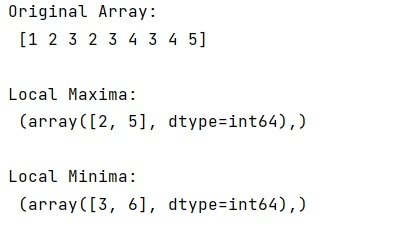# Finding local maxima/minima with NumPy in a 1D NumPy array

Learn how to find local maxima/minima with NumPy in a 1D NumPy array?
Submitted by Pranit Sharma, on December 24, 2022

NumPy is an abbreviated form of Numerical Python. It is used for different types of scientific operations in python. Numpy is a vast library in python which is used for almost every kind of scientific or mathematical operation. It is itself an array which is a collection of various methods and functions for processing the arrays.

## Finding local maxima/minima with a 1D NumPy array

Suppose that we are given a one-dimensional NumPy array and we need to develop an approach to find local maxima and local minima in this NumPy array.

Mathematically, if f is the function defined in an open interval I. And f be continuous at critical point c in I such that f'(c) = 0.

1. If f'(x) changes sign from positive to negative as x increases through point c, then c is the point of local maxima. And the f(c) is the maximum value.
2. If f'(x) changes sign from negative to positive as x increases through point c, then c is the point of local minima. And the f(c) is the minimum value.

For this purpose, we will use the argrelextrema() method from the SciPy signal module. For finding local maxima, we will pass np.greater as an argument and np.less for local minima.

Let us understand with the help of an example,

## Python code to find local maxima/minima with NumPy in a 1D NumPy array

```# Import numpy
import numpy as np

# Import argreletextrema
from scipy.signal import argrelextrema

# Creating a numpy array
arr = np.array([1,2,3,2,3,4,3,4,5])

# Display original array
print("Original Array:\n",arr,"\n")

# Finding local maxima
max = argrelextrema(arr,np.greater)

# Display Local maxima
print("Local Maxima:\n",max,"\n")

# Finding local minima
min = argrelextrema(arr,np.less)

# Display Local minima
print("Local Minima:\n",min,"\n")
```

Output: